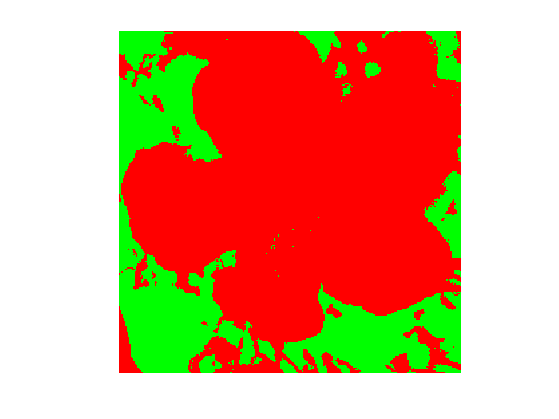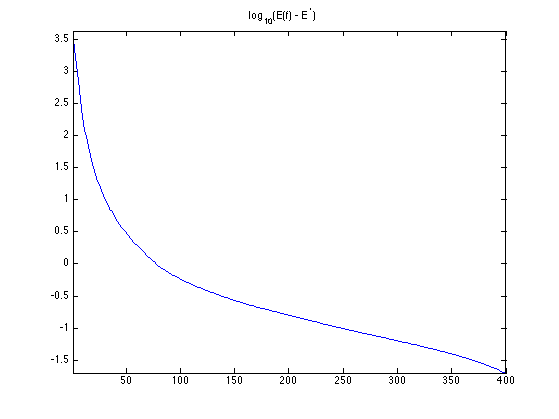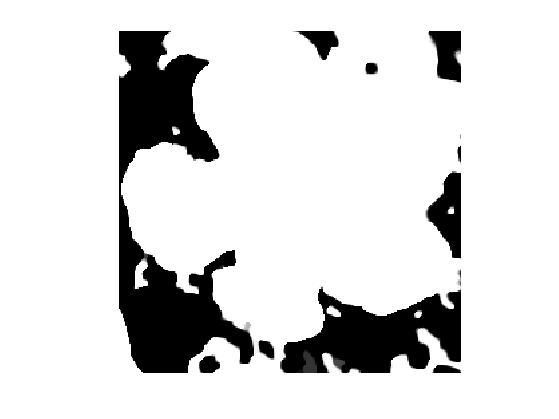$\newcommand{\NN}{\mathbb{N}} \newcommand{\CC}{\mathbb{C}} \newcommand{\GG}{\mathbb{G}} \newcommand{\LL}{\mathbb{L}} \newcommand{\PP}{\mathbb{P}} \newcommand{\QQ}{\mathbb{Q}} \newcommand{\RR}{\mathbb{R}} \newcommand{\VV}{\mathbb{V}} \newcommand{\ZZ}{\mathbb{Z}} \newcommand{\FF}{\mathbb{F}} \newcommand{\KK}{\mathbb{K}} \newcommand{\UU}{\mathbb{U}} \newcommand{\EE}{\mathbb{E}} \newcommand{\Aa}{\mathcal{A}} \newcommand{\Bb}{\mathcal{B}} \newcommand{\Cc}{\mathcal{C}} \newcommand{\Dd}{\mathcal{D}} \newcommand{\Ee}{\mathcal{E}} \newcommand{\Ff}{\mathcal{F}} \newcommand{\Gg}{\mathcal{G}} \newcommand{\Hh}{\mathcal{H}} \newcommand{\Ii}{\mathcal{I}} \newcommand{\Jj}{\mathcal{J}} \newcommand{\Kk}{\mathcal{K}} \newcommand{\Ll}{\mathcal{L}} \newcommand{\Mm}{\mathcal{M}} \newcommand{\Nn}{\mathcal{N}} \newcommand{\Oo}{\mathcal{O}} \newcommand{\Pp}{\mathcal{P}} \newcommand{\Qq}{\mathcal{Q}} \newcommand{\Rr}{\mathcal{R}} \newcommand{\Ss}{\mathcal{S}} \newcommand{\Tt}{\mathcal{T}} \newcommand{\Uu}{\mathcal{U}} \newcommand{\Vv}{\mathcal{V}} \newcommand{\Ww}{\mathcal{W}} \newcommand{\Xx}{\mathcal{X}} \newcommand{\Yy}{\mathcal{Y}} \newcommand{\Zz}{\mathcal{Z}} \newcommand{\al}{\alpha} \newcommand{\la}{\lambda} \newcommand{\ga}{\gamma} \newcommand{\Ga}{\Gamma} \newcommand{\La}{\Lambda} \newcommand{\Si}{\Sigma} \newcommand{\si}{\sigma} \newcommand{\be}{\beta} \newcommand{\de}{\delta} \newcommand{\De}{\Delta} \renewcommand{\phi}{\varphi} \renewcommand{\th}{\theta} \newcommand{\om}{\omega} \newcommand{\Om}{\Omega} \renewcommand{\epsilon}{\varepsilon} \newcommand{\Calpha}{\mathrm{C}^\al} \newcommand{\Cbeta}{\mathrm{C}^\be} \newcommand{\Cal}{\text{C}^\al} \newcommand{\Cdeux}{\text{C}^{2}} \newcommand{\Cun}{\text{C}^{1}} \newcommand{\Calt}{\text{C}^{#1}} \newcommand{\lun}{\ell^1} \newcommand{\ldeux}{\ell^2} \newcommand{\linf}{\ell^\infty} \newcommand{\ldeuxj}{{\ldeux_j}} \newcommand{\Lun}{\text{\upshape L}^1} \newcommand{\Ldeux}{\text{\upshape L}^2} \newcommand{\Lp}{\text{\upshape L}^p} \newcommand{\Lq}{\text{\upshape L}^q} \newcommand{\Linf}{\text{\upshape L}^\infty} \newcommand{\lzero}{\ell^0} \newcommand{\lp}{\ell^p} \renewcommand{\d}{\ins{d}} \newcommand{\Grad}{\text{Grad}} \newcommand{\grad}{\text{grad}} \renewcommand{\div}{\text{div}} \newcommand{\diag}{\text{diag}} \newcommand{\pd}{ \frac{ \partial #1}{\partial #2} } \newcommand{\pdd}{ \frac{ \partial^2 #1}{\partial #2^2} } \newcommand{\dotp}{\langle #1,\,#2\rangle} \newcommand{\norm}{|\!| #1 |\!|} \newcommand{\normi}{\norm{#1}_{\infty}} \newcommand{\normu}{\norm{#1}_{1}} \newcommand{\normz}{\norm{#1}_{0}} \newcommand{\abs}{\vert #1 \vert} \newcommand{\argmin}{\text{argmin}} \newcommand{\argmax}{\text{argmax}} \newcommand{\uargmin}{\underset{#1}{\argmin}\;} \newcommand{\uargmax}{\underset{#1}{\argmax}\;} \newcommand{\umin}{\underset{#1}{\min}\;} \newcommand{\umax}{\underset{#1}{\max}\;} \newcommand{\pa}{\left( #1 \right)} \newcommand{\choice}{ \left\{ \begin{array}{l} #1 \end{array} \right. } \newcommand{\enscond}{ \left\{ #1 \;:\; #2 \right\} } \newcommand{\qandq}{ \quad \text{and} \quad } \newcommand{\qqandqq}{ \qquad \text{and} \qquad } \newcommand{\qifq}{ \quad \text{if} \quad } \newcommand{\qqifqq}{ \qquad \text{if} \qquad } \newcommand{\qwhereq}{ \quad \text{where} \quad } \newcommand{\qqwhereqq}{ \qquad \text{where} \qquad } \newcommand{\qwithq}{ \quad \text{with} \quad } \newcommand{\qqwithqq}{ \qquad \text{with} \qquad } \newcommand{\qforq}{ \quad \text{for} \quad } \newcommand{\qqforqq}{ \qquad \text{for} \qquad } \newcommand{\qqsinceqq}{ \qquad \text{since} \qquad } \newcommand{\qsinceq}{ \quad \text{since} \quad } \newcommand{\qarrq}{\quad\Longrightarrow\quad} \newcommand{\qqarrqq}{\quad\Longrightarrow\quad} \newcommand{\qiffq}{\quad\Longleftrightarrow\quad} \newcommand{\qqiffqq}{\qquad\Longleftrightarrow\qquad} \newcommand{\qsubjq}{ \quad \text{subject to} \quad } \newcommand{\qqsubjqq}{ \qquad \text{subject to} \qquad }$

Convex Region-Based Image Segmentation

# Convex Region-Based Image Segmentation

This numerical tour explores a convex relaxation of the piecewise constant Mumford-Shah. This relaxation is exact, and leads to a global solution to the segmentation problem. It can be solved using proximal splitting scheme, and we propose to use here the Douglas-Rachford algorithm. Of independent interest is the introduction of auxiliary gradient variables that enables the use of purely primal splitting schemes.

## Contents

Special thanks to Jalal Fadili for telling me about the "auxiliary variable" trick (i.e. adding the variable $$u=\nabla f$$), that allows one to solve TV regularization without the need to use primal-dual schemes.

## Installing toolboxes and setting up the path.

You need to download the following files: signal toolbox and general toolbox.

You need to unzip these toolboxes in your working directory, so that you have toolbox_signal and toolbox_general in your directory.

For Scilab user: you must replace the Matlab comment '%' by its Scilab counterpart '//'.

Recommandation: You should create a text file named for instance numericaltour.sce (in Scilab) or numericaltour.m (in Matlab) to write all the Scilab/Matlab command you want to execute. Then, simply run exec('numericaltour.sce'); (in Scilab) or numericaltour; (in Matlab) to run the commands.

Execute this line only if you are using Matlab.

getd = @(p)path(p,path); % scilab users must *not* execute this


Then you can add the toolboxes to the path.

getd('toolbox_signal/');
getd('toolbox_general/');


## Binary Segmentation

We consider some input image $$I(x) \in \RR^d$$ ($$d=1$$ for grayscale image and $$d=3$$ for color images). Given weights $$w_0$$ and $$w_1$$ computed from $$I$$, where $$N$$ is the number of pixel, the goal is to find a region $$\Om$$ that minimize $\umin{\Om} \int_\Om w_0(x) d x + \int_{\Om^c} w_1(x) d x + \la \abs{\partial \Om},$ where $$\abs{\partial \Om}$$ is the perimeter of $$\Om$$.

To perform region based piecewise-constant segmentation, we assume we know the target values $$c_0, c_1 \in \RR^d$$ for the inside/outside of the segmented domain, and use quadratic weigths $\text{for } i=0,1, \quad w_i(x) = \norm{I(x)-c_i}^2.$ We use these weights in all the remaining part of this tour.

In the special case $$\la=0$$, no regularization is performed, and the optimal set  is obtained by a simple thresholding $\Om = \enscond{x}{ w_0(x) \leq w_1(x). }$

We load the image $$I$$.

name = 'hibiscus';
n = 256;


Display it.

clf;
imageplot(I);Take as target colors red and green.

c0 = [1;0;0];
c1 = [0;1;0];


Exercice 1: (check the solution) Compute $$w_0$$ and $$w_1$$. Compute and display the segmentation when $$\la=0$$.

exo1;Define $$w=w_0-w_1$$.

w = w0-w1;


## Convex Discrete Formulation

If one represents $$\Om$$ using its indicator function $$f$$ $f(x) = \chi_\Om(x) = \choice{ 1 \qifq x \in \Om. \\ 0 \quad \text{otherwise}, }$ this problem is re-casted equivalently $\umin{ f(x) \in \{0,1\} } \dotp{f}{w} + \la \norm{f}_{\text{TV}},$ where $$w=w_0-w_1$$ and $$\norm{f}_{\text{TV}}$$ is the total variation pseudo-norm, that is equal to $$\abs{\partial \Om}$$ for binary indicator $$f=\chi_\Om$$. Here the inner product is the canonical one $$\dotp{f}{w}=\int f w$$.

The variational problem is discretized on a grid of $$N=n \times n$$ pixels, and we define the total variation pseudo-norm, for $$f \in \RR^N$$, as $\norm{f}_{\text{TV}} = \norm{\nabla f}_1 \qwhereq \norm{u}_1 = \sum_{i=1}^N \norm{u_i},$ when $$u=(u_i)_{i=1}^N \in \RR^{N \times 2}, u_i \in \RR^2$$ is a vector field.

We use a finite difference gradient operator $(\nabla f)_i = (f_{i+\de_1}-f_i, f_{i+\de_2}-f_i) \in \RR^2,$ (we assume the pixels are indexed on a 2-D grid) where $$\de_1=(1,0)$$ and $$\de_2=(0,1)$$. We use periodic boundary conditions for simplicity.

options.bound = 'per';
Div = @(x)div(x,options);


The inner product in the objective is discretized using the canonical inner product in $$\RR^N$$ $\dotp{f}{w} = \sum_{i=1}^N f_i w_i .$

To obtain a convex program, one replaces the binary constraint $$f_i \in \{0,1\}$$ by a box constraint $$f_i \in [0,1]$$. This defines the folowing finite dimensional convex problem $\umin{ f \in [0,1]^N } \dotp{f}{w} + \la \norm{\nabla f}_{1}.$

One can prove that this relaxation is exact, meaning that the minimizer $$f$$, when it is unique, is binary, $$f \in \{0,1\}^N$$. It means that $$\Om$$ such that $$f=\chi_\Om$$ actually solves the original segmentation problem. See for instance:

Tony F. Chan, Selim Esedoglu, and Mila Nikolova. Algorithms for finding global minimizers of image segmentation and denoising models SIAM J. Appl. Math., 66(5):1632-1648, 2006.

It is possible to generalize this convexification method to the segmentation problem with more than 2 partitions. See for instance:

Antonin Chambolle, Daniel Cremers, Thomas Pock, A convex approach to minimal partitions, Preprint hal-00630947, 2011.

To solve this problem using primal proximal splitting scheme, we introduce an auxiliary variable $$u=\nabla f$$, and write the optimization problem as $\umin{z=(f,u) \in \Zz = \RR^N \times \RR^{N \times 2} } F(z) + G(z) \qwhereq \choice{ F(f,u) = \dotp{f}{w} + \iota_{[0,1]^N}(f) + \la \norm{u}_1, \\ G(f,u) = \iota_{\Cc}(f,u), }$ where here we included the constraints using indicator functions $\iota_{A}(z) = \choice{ 0 \qifq z \in A, \\ +\infty \quad \text{otherwise}. }$ The constraint linking $$f$$ to $$u$$ is $\Cc = \enscond{z = (f,u) \in \Zz}{ u=\nabla f }.$

## Douglas-Rachford Algorithm

To minimize the segmentation energy, we will make use of proximal splitting scheme. These scheme are adapted to solve structured non-smooth optimization problem.

They basically replace the traditional gradient-descent step (that is not available because neither $$F$$ nor $$G$$ are smooth functionals) by proximal mappings, defined as $\text{Prox}_{\gamma F}(z) = \uargmin{y} \frac{1}{2}\norm{z-y}^2 + \ga F(y)$ (the same definition applies also for $$G$$).

The Douglas-Rachford (DR) algorithm is an iterative scheme to minimize functionals of the form $\umin{z} F(z) + G(z)$ where $$F$$ and $$G$$ are convex functions for which one is able to comptue the proximal mappings $$\text{Prox}_{\gamma F}$$ and $$\text{Prox}_{\gamma G}$$.

The important point is that $$F$$ and $$G$$ do not need to be smooth. One onely needs then to be "proximable".

A DR iteration reads $\tilde z_{k+1} = \pa{1-\frac{\mu}{2}} \tilde z_k + \frac{\mu}{2} \text{rPox}_{\gamma G}( \text{rProx}_{\gamma F}(\tilde z_k) ) \qandq z_{k+1} = \text{Prox}_{\gamma F}(\tilde z_{k+1},)$

We have use the following shortcuts: $\text{rProx}_{\gamma F}(z) = 2\text{Prox}_{\gamma F}(z)-z$

It is of course possible to inter-change the roles of $$F$$ and $$G$$, which defines another set of iterations.

One can show that for any value of $$\gamma>0$$, any $$0 < \mu < 2$$, and any $$\tilde z_0$$, $$z_k \rightarrow z^\star$$ which is a minimizer of $$F+G$$.

Please note that it is actually $$z_k$$ that converges, and not $$\tilde z_k$$.

Proximal Splitting Methods in Signal Processing, Patrick L. Combettes and Jean-Christophe Pesquet, in: Fixed-Point Algorithms for Inverse Problems in Science and Engineering, New York: Springer-Verlag, 2010.

## Proximal Operator of $$G$$

The proximal mapping of $$G$$ is the orthogonal projection on the convex set $$G$$: $(\tilde f, \tilde u) = \text{Prox}_{\ga G}(f,u) = \text{Proj}_\Cc(f,u).$

It can be computed by solving a linear system of equations since $\tilde u = \nabla \tilde f \qwhereq \tilde f = (\text{Id}_N - \Delta)^{-1}(f-\text{div}(u)).$ Here, by convention, $$\Delta=\text{div} \circ \nabla$$ and $$\text{div}=-\nabla^*$$.

Since we use periodic boundary conditions for the gradient operator, it is possible to solve this linear system in $$O(N \log(N))$$ operations using the FFT algorithm. Note that a similar method can be used with non-periodic Neumann condition (this requires to extend by symmetry the image).

One indeed has $\forall \om=(\om_1,\om_2), \quad \hat {\tilde f}(\om) = \frac{\hat g(\om)}{K(\om)} \qwhereq K(\om) = 1+4\sin\pa{\frac{\pi \om_1}{n}}^2+4\sin\pa{\frac{\pi \om_2}{n}}^2,$ where $$g = f-\text{div}(u)$$ and where $$\hat g$$ is the 2-D discrete Fourier transform of an image $$g$$.

Compute $$K(\om)$$.

[X Y] = meshgrid(0:n-1, 0:n-1);
K = 1 + 4*sin(X*pi/n).^2 + 4*sin(Y*pi/n).^2;


Define Proj$$_\Cc$$.

Replicate = @(z)deal(z, Grad(z));
ProjC = @(f,u)Replicate( real( ifft2( fft2( f - Div(u) ) ./ K ) ) );


One has $$\text{Prox}_{\ga G} = \text{Proj}_{\Cc}$$, whatever the value of $$\ga$$.

ProxG = @(f,u,gamma)ProjC(f,u);


## Proximal Operator of $$F$$

Recall that the function $$F(f,u)$$ is actully a separable sum of a function that only depends on $$f$$ and a function that depends only on $$u$$: $F(f,u) = F_0(f) + \la \norm{u}_1 \qwhereq F_0(f) = \dotp{f}{w} + \iota_{[0,1]^N}(f)$ The proximal operator of $$F$$ reads $\text{Prox}_{\ga F}(f,u) = ( \text{Prox}_{\ga F_0 }(f), \text{Prox}_{\ga \la \norm{\cdot}_1 }(u) ).$

Define the value of $$\la>0$$ (you can change this value).

lambda = .1;


The proximal operator of $$F_0$$ is obtained by using the projection on the box constraint $\text{Prox}_{\ga F_0 }(f) = \text{Proj}_{[0,1]^N}( f - \ga w ) \qwhereq \text{Proj}_{[0,1]^N}(g) = \max(0,\min(1, g)).$

ProxF0 = @(f,gamma)max(0, min(1, f-gamma*w)  );


The proximal operator of the $$\ell^1-\ell^2$$ norm $$\norm{\cdot}_1$$ is a soft thresholding of the amplitude of the vector field: $\text{Prox}_{\ga \norm{\cdot}_1}(u)_i = \max\pa{ 0, \frac{\ga}{\norm{u_i}} } u_i.$

amplitude = @(u)repmat( sqrt( sum(u.^2, 3) ), [1 1 2]);
ProxL1 = @(u,gamma)max(0,1-gamma./max(1e-9, amplitude(u))) .* u;


Define the proximal operator of $$F$$.

ProxF = @(f,u,gamma)deal( ProxF0(f,gamma), ProxL1(u,gamma*lambda) );


## Douglas-Rachford for Convex Segmentation

Set the value of $$\mu$$ and $$\gamma$$. You might consider using your own value to speed up the convergence.

mu = 1;
gamma = 1;


Number of iterations.

niter = 800;


Exercice 2: (check the solution) Implement the DR iterative algorithm on niter iterations. Keep track of the evolution of the minimized energy $E(f) = \dotp{w}{f} + \la \norm{\nabla f}_1$ during the iterations. Remark: to speedup the convergence, you can use a "clever" initialization.

exo2;Display the result image $$f$$ at convergence. Note that $$f$$ is almost binary.

clf;
imageplot(f);Exercice 3: (check the solution) Test with different value of the $$\lambda$$ parameter.

exo3;